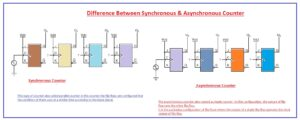Hello, friends welcome to the new post. In this post, we will cover Difference Between Synchronous & Asynchronous Counter. In field of digital electronics, the counter is a logic circuitry that creates through the use of flip flops connected in series combinationss. It used to do counting of quantity of operations of input terminology of negative and positive corner variants. There common applications for synchronization and frequency calculation.

There some features is like the registers since these two modules are consist of flip-flops. There are 2 main types of counter first one is synchronous and the second one is asynchronous counters. The basic difference is that in synchronous counter all flip flops get triggered through the use of a single clock and in asynchronous counter there is different clock are used.

So let’s get started.

## Difference Between Synchronous & Asynchronous Counter

Asynchronous Counter

• The asynchronous counter also named as ripple counter. In this configuration, the output of flip flop runs the other flip flop.
• It is the packaging configuration of flip flops where the output of a single flip flop operates the clock output of flip flop.
• The ripple counter comprises of a sequence of a flip flops here the output of every flip flop is linked to the clock input of the subsequent flip flop.
• The signal is given to the ist flip flop and then moves to the next having a time delay.
• For example, the output of ist flip flop is the clock input of the 2nd flip flop, and the output of 2nd flip flop is the signal input of 3rd flip flop.
• Its common used type are four-bit up counter, four it down the counter and four-bit up/down counterSynchronous Counter

• This type of counter also called parallel counter in this counter the flip flops are configured that the condition of them vary at a similar time according to the input signal.
• If the pulse gave is such tath every flip flop in the counter gets triggered through a single pulse due to that it is known as a synchronous counter.
• Contrary to the asynchronous counter the counter input is linked to the inputs of every flip flop. Since every flip flop gets a pulse at a single time due to that cause these counter can operate at high speed than the asynchronous counter.What is Synchronous Counter

• It also named as a synchronous counter.
• Its configuration is that in this module all flip flops get triggered with a similar clock pulse.
• Its design is somewhat complicated.
• The sequence for this module is fixed.
• In this arrangement, there is no fault due to the decoding.
• Its functioning speed is high than other counters.
• According to the clock signal, every flip flops its state at the same time.
• It employed in clocks

What is Asyncrhnous Counter

• It named a serial counter.
• In this module, there is a separate pulse needed for each flip flop.
• Its practical use is frequency divider circuits
• There is a decoding fault that exists in this device.
• its functioning speed is less than other devices.
• Its flip flop does not vary in condition according to the resultant pulse.

That is a detailed post about Difference Between Synchronous & Asynchronous Counter. I tried to cover each and every parameter related to this if you have any further query ask in the comments. Thanks for reading.